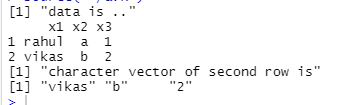Open in App
Not now

# How to convert a DataFrame row into character vector in R?

• Last Updated : 21 Apr, 2021

If we want to turn a dataframe row into a character vector then we can use as.character() method In R, we can construct a character vector by enclosing the vector values in double quotation marks, but if we want to create a character vector from data frame row values, we can use the as character function.
For example, if we have a data frame df then the values in the first row of the df can be turned into character vector using as.character(df[1,]).

Syntax: as.character(df[rownum, ])

Example 1: Here we are going to turn dataframe row into a character vector.

Here we will create a dataframe and then convert it to a vector.

## R

 `# creating dataframe``data <- ``data.frame``(x1 = 1:5,              ``                  ``x2 = ``letters``[1:5],``                  ``x3 = 6:10)`` ` `# converting first row into character vector``first_row_vector = ``as.character``(data[1, ]);`` ` `print``(first_row_vector)`

Output:

` "1" "1" "6"`

Example 2: Converting row-wise dataframe into the vector.

## R

 `data <- ``data.frame``(x1 = ``c``(``"rahul"``, ``"vikas"``) ,            ``                  ``x2 = ``letters``[1:2],``                  ``x3 = ``c``(1,2))`` ` `first_row_vector = ``as.character``(data[2, ]);`` ` `print``(``"dataFrame"``)``print``(data)`` ` `print``(``"character vector of first row is"` `)``print``(first_row_vector)`

Output:My Personal Notes arrow_drop_up